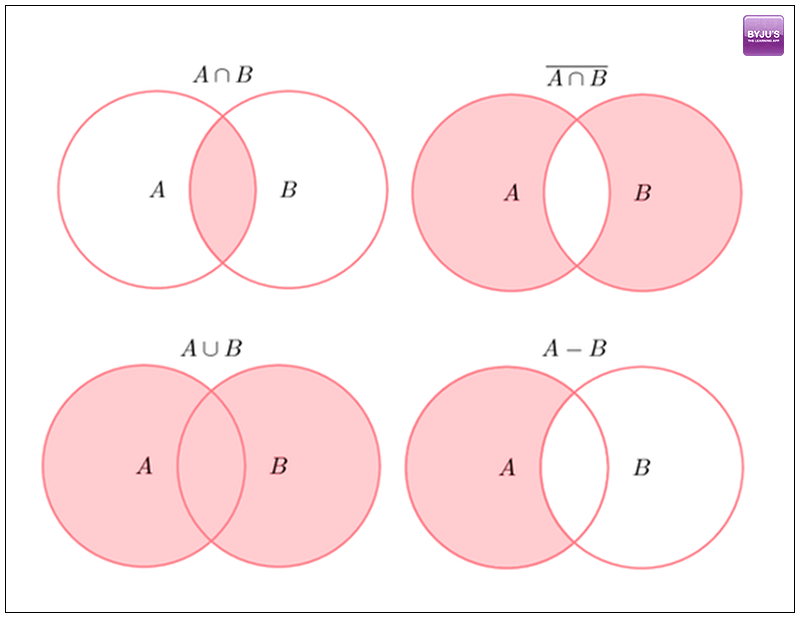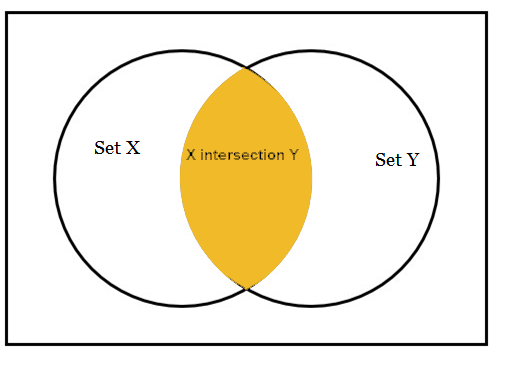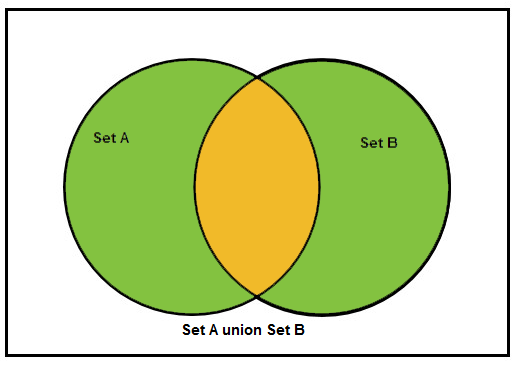# GMAT Quant: Sets and Venn DiagramsSets are the most fundamental concepts of mathematics. It is a collection of elements or objects. If the member of the sets are just numbers, then the ‘set notation’ is used which is comprised of brackets.

Such as, R = {1, 2, 3, 4, 5, 6, 7, 8}; where R is the set that contains eight members that are numbers.

The favorite topic of GMAT in the section of sets is overlapping sets. Click here to read more on GMAT Sets.

An overlapping set is a set that shares it’s boundaries with two or more different sets. The simplest form of overlapping set is a Venn diagram, depicting two overlapping circles.When two sets shares some common properties, these properties are said to be overlapping.

Set operations:

## Intersection of Two Sets:

X Y## Overlapping of Two Sets:

A BQuestion: Out of forty students, 14 are taking English composition and 29 are taking Chemistry. How many students are in neither class?

1. Maximum of 10 students out of 40 can take both classes.
2. 5 students are in both classes.

Decide whether the data given are sufficient for answering the question and then indicate one of the following answer choices:

1. Statement (1) ALONE is sufficient, but statement (2) alone is not sufficient.
2. Statement (2) ALONE is sufficient, but statement (1) alone is not sufficient.
3. BOTH statements TOGETHER are sufficient, but neither statement ALONE is sufficient.
4. EACH statement ALONE is sufficient.
5. Statement (1) and (2) TOGETHER are not sufficient.

Solution:

Statement (1): Given maximum of 10 students out of 40 can take both classes, but for finding number of students who are in neither class, we need to know the exact number of students taking both classes.

NOT sufficient.

Statement (2): 5 students are in both classes.

So, number of students who are in neither classes = 40-14-29+5 = 2

Sufficient.

Question: Many of the students at Oxford University speak English or German or both. Among the students who speak English, four times as many speak German as don’t. In addition, 1/6 of the students who don’t speak German do speak English. What fraction of the students speak German?

1. Exactly 60 students speak English and German.
2. Exactly 75 students speak neither English nor German.

Decide whether the data given are sufficient for answering the question and then indicate one of the following answer choices:

1. Statement (1) ALONE is sufficient, but statement (2) alone is not sufficient.
2. Statement (2) ALONE is sufficient, but statement (1) alone is not sufficient.
3. BOTH statements TOGETHER are sufficient, but neither statement ALONE is sufficient.
4. EACH statement ALONE is sufficient.
5. Statement (1) and (2) TOGETHER are not sufficient.

Solution:

 English No English German 4x (?) No German X 5x 6x 6x

Statement 1:- 4x = 60

x = 15

So, the number of students speaking German = 180+60 = 240

No. of students not speaking German = 6

NOT sufficient.

Statement 1 and 2:- Using both statement together also we can get only x = 15 and not the total fraction of students who speak German.

Not sufficient.